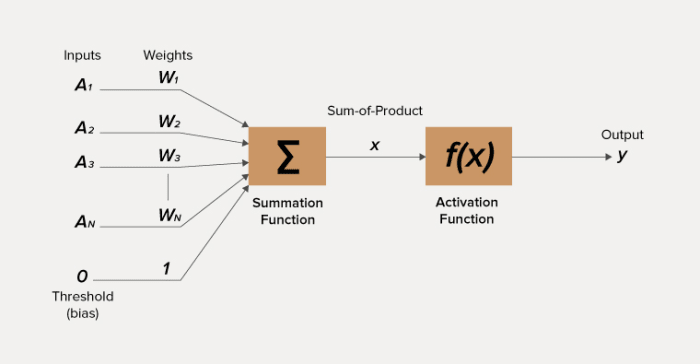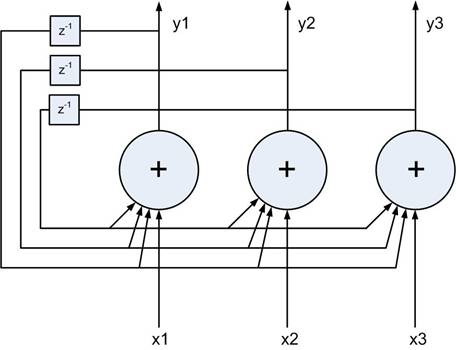# How to start a neural network with JavaScript in 5 minutes

Software development is advancing incredibly fast these days and neural networks are becoming increasingly important. You may not even be aware of the role they already play in everyday life; in houses, cars, and workplaces.Kamil Mikosz

Frontend Developer

The question is: can a JavaScript developer, aware of the pace of progress and the complexity of this technology, start creating simple or even advanced neural networks? Especially if he or she has never had anything to do with them before, specializing instead in web applications?

The answer is YES. In this article I’m going to show you how to create a simple network using JavaScript in just 5 minutes.

## A brief theoretical introduction

A little bit of theory will be of use before you begin your adventure with neural networks – this will help you not only to create a working network but also to be familiar with the way it functions.

The neuron is both the most important and the smallest element in the network structure. It’s a simple input/output function, processing multiple input signals into a single output signal. The way the signal is converted is determined by the activation function (e.g. step, sigmoid, or linear), the signal weight, and the bias.

The bias is a signal whose weight always equals one, which allows the adjustment of the activation function (moving to the left or right).

Graphically, an individual neuron can be presented this way:source: http://www.ece.utep.edu

To better illustrate the way a neuron functions, I’ll try to determine its output on the example of some example data. Our example neuron has three input signals – one of them is the bias (value: 0.5), the weights of the others are 0.4 and 0.5 respectively. I’m going to use the unit step (for values higher than 0, the value of the signal is 1) as an activation function.

If both of the inputs (leaving out the bias) have a signal whose value is 1, the output of the neuron can be calculated in the following manner:

y = f(0.5*1 + 0.4*1 – 0.5) = f (0.4) = 1

Now that I have the basic network unit I can take the next step – that is, create a layer from it and adjust the network to a particular problem. A layer is a sequence of connected neurons which can transmit signals from one to another There are three kinds of network layers: input, output, and hidden. The input and output layers only have one level each, while the hidden layer can have multiple levels.

Graphically, our example network can be presented this way:These are the basic elements of a simple neural network. In this article, I want to go even further and show you a more interesting and practical example. To do that, the notion of the Hopfield network must be introduced. It can be illustrated this way:source: http://galaxy.agh.edu.pl

These are the characteristic features of the Hopfield network:

• Feedback (any time you calculate the value of the neuron, you must take the value calculated in the previous cycle into account),
• The neuron value calculated in one iteration is transmitted to all other neurons,
• If the initial neuron weights are zeroed out, the network acts as associative memory,
• There is only one layer of neurons.

In practice, the network will learn the patterns based on the provided data, adjusting the neuron weights by means of adequate rules. If you’d like to know more on this subject, have a look at this article.

Examples of how a Hopfield network can be used include pattern recognition, classification, and process optimization.

## Implementing a simple neural network

Now that you know the basics, it’s time to get down to work. I’d like to show how easy it is to get from theory to practice. The test project consists in creating a simple dictionary containing three words and training the network so that it could recognize the words even if they contain small deformations. To do it, create a .js script and run it with Node. Node is a tool to start JavaScript on the server.

To begin, let’s create a catalogue for the project – in my case it’s called “Hopfield network”. Open the project in the terminal and initiate it through the command:

– npm init

The command initiates the project by creating the package.json file and saving the project details there. To start the project and download packages, you can also use yarn.

Next, download the latest version of SynapticJS. This is a library that lets you design, train, and use neural networks; use the command:

-npm install synaptic –save

Now everything is ready, you can start working with the network. Create the index.js to store the logic of the project.

After creating the project, add the library and create some constants:

``````// Add synaptic library
const synaptic = require('synaptic');

// Create dictionary as our source of knowledge
const dictionary = [
'cat',
'bob',
'ice',
//...
];

// Define binary size for one letter
const binarySize = 7;

// Define number of neurons in our network. It's word length multiplied by binarySize
const neuronsCount = 21;``````

In the code above:

• The dependency of the synapticJS library has been created,
• A simple dictionary of words used to train the network has been created; in this example, it contains only three words – in practice, the set training neural networks would be much larger,
• The binary size for one letter has been defined – this refers to the number of binary characters included in one letter after transformation into a binary string, resulting from the size of the binary string according to the ASCII character table (max. 128 characters in the basic version),
• The number of neurons in the network has been established – it can be defined as the product of the number of binary characters in one letter and the number of letters; for the purposes of this example, let’s assume that the learning set can only contain three-letter words.
• Then, two auxiliary functions are created to help translate the words into a binary string, which is the form understood by the neural network, and to transform the binary string into a single word.

Then, two auxiliary functions are created to help translate the words into a binary string, which is the form understood by the neural network, and to transform the binary string into a single word.

``````// Transform word to binary string
const wordToBinary = function (word) {
let binaryWord = '';

for (let i = 0; i < word.length; i++) {
binaryWord += word.charCodeAt(i).toString(2);
}

return binaryWord;
};

// Transform binary string to word
const binaryToWord = function (binaryWord) {
const wordLength = binaryWord.length / binarySize;
let word = '';

for (let i = 0; i < wordLength; i++) {
word += String.fromCharCode(parseInt(binaryWord.slice(i * binarySize, i * binarySize + binarySize).join(''), 2));
}

return word;
};``````

Now you can move on to implementing the neural network:

``````// Create Hopfield Neural Network
const hopfieldNetwork = new synaptic.Architect.Hopfield(neuronsCount);

// Create dictionary of binary words
const binaryDicitonary = dictionary.map(word => wordToBinary(word));

// Learn our network new patterns
hopfieldNetwork.learn(binaryDicitonary);

// Let's check if it works
console.log(binaryToWord(hopfieldNetwork.feed(wordToBinary('cob'))));``````

At the beginning, create the network using the architect provided by the SynapticJS library – you could design the structure of the network yourself, but that can take a lot of time. Next, transform the dictionary into a binary string and train the network. In the last line, the result of the neural network prediction for the word ‘cob’ is checked.

Now the app is ready to open. This can be done by this call:

`node index.js`

You can see that the terminal shows the word ‘bob’. Why is that? The neural network knows three patterns defined in the dictionary. When the word ‘cob’ was entered in the network, it iterated until it encountered one of the patterns it remembered (it happens sometimes that the network recognizes a spurious state). The designated pattern was then transformed from the binary form – readable for the network – into a form understandable for you: a word.

If you want to become more familiar with the algorithms of learning, teaching, and finding patterns, take a look here.

## Summary

Just like I promised at the beginning, you need no more than 5 minutes to create a functioning neural network that can guess words with small typos. It must be mentioned, however, that the Hopfield network has some disadvantages and is not able to guarantee the recognition of a proper pattern each time. This is caused by the fact that sometimes an erroneous local minimum is found which reflects a false pattern.

So it turns out that the basic issues related to neural networks are not as overwhelming as you might expect. The Hopfield network is just one of many that can be used nowadays. In practice of course, more complicated structures are employed, sometimes combining more than one algorithm.

Last but not least, I’d like to encourage you to take a closer look at the SynapticJS library as well as at other libraries that enable you to create and use neural networks by means of JavaScript:

Contact: